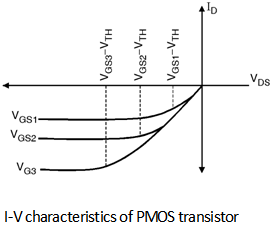Home > Analog CMOS Design > MOSFET Fundamentals > I V Characteristics of PMOS Transistor

I-V Characteristics of PMOS Transistor :

In order to obtain the relationship between the drain to source current (I DS) and its terminal voltages we divide characteristics in two regions of operation i.e. linear region and saturation region.

In linear region the IDS will increase linearly with increase in drain to source voltage (VDS) whereas in saturation region the I DS is constant and it is independent of VDS.

The relationship between the source to drain current (ISD) and its terminal voltages can be derived by the same procedure as that of the NMOS transistor. Only the change is in this case the carriers present in the inversion layer are holes and as hole moves from source to drain the current flow is also in the same direction as that of the hole.

Hence -ve sign appears in the current equation. Also all the biases applied at the device terminals are -ve. The ID - VDS characteristics of PMOS transistor are shown inFigure belowFor PMOS device the drain current equation in linear region is given as :

ID = - mp Cox

Similarly the Drain current equation in saturation region is given as :

ID = - mp Cox (VSG - | V TH |p )2

Where mp is the mobility of hole and |VTH| p is the threshold voltage of the PMOS transistor.

The negative sign appeared in the equation of I D shows that I D flows from drain to the source where as holes flow in the reverse direction. As the mobility of hole is less than the mobility of electron PMOS devices suffer from the lower current drive capability compare to NMOS devices.